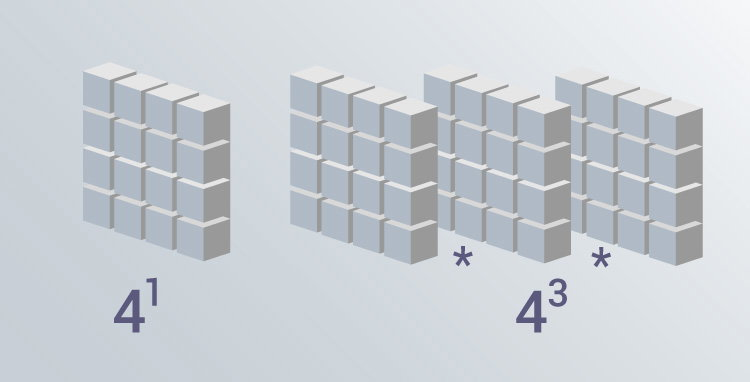# C Program to Calculate the Power of a Number

Example on how to calculate the power of a number if the exponent is an integer. Also, you will learn to compute the power using pow() function.To understand this example, you should have the knowledge of following C programming topics:

The program below takes two integers from the user (a base number and an exponent) and calculates the power.

For example: In case of 23

• 2 is the base number
• 3 is the exponent
• And, the power is equal to 2*2*2

## Example #1: Power of a Number Using while Loop

``````#include <stdio.h>
int main()
{
int base, exponent;

long long result = 1;

printf("Enter a base number: ");
scanf("%d", &base);

printf("Enter an exponent: ");
scanf("%d", &exponent);

while (exponent != 0)
{
result *= base;
--exponent;
}

return 0;
}
``````

Output

```Enter a base number: 3
Enter an exponent: 4
```

The above technique works only if the exponent is a positive integer.

If you need to find the power of a number with any real number as an exponent, you can use pow() function

## Example #2: Power Using pow() Function

``````#include <stdio.h>
#include <math.h>

int main()
{
double base, exponent, result;

printf("Enter a base number: ");
scanf("%lf", &base);

printf("Enter an exponent: ");
scanf("%lf", &exponent);

// calculates the power
result = pow(base, exponent);

printf("%.1lf^%.1lf = %.2lf", base, exponent, result);

return 0;
}
``````

Output

```Enter a base number: 2.3
Enter an exponent: 4.5
2.3^4.5 = 42.44```